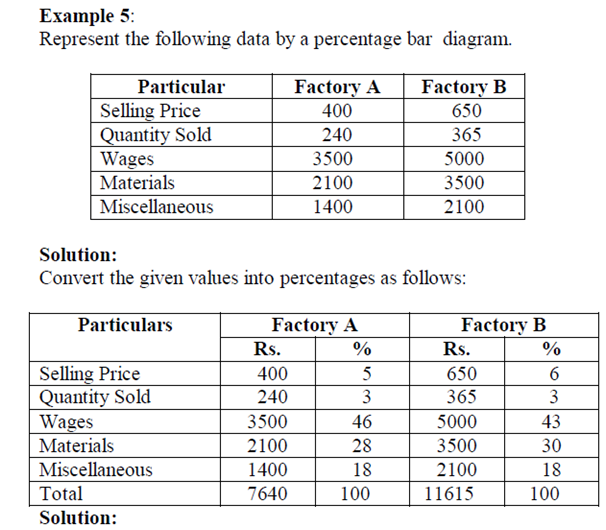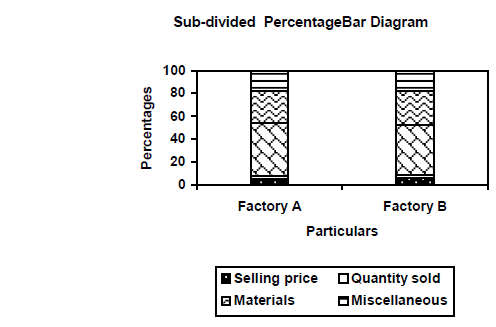# Statistics for SSC-CGL Tier-II (Paper-III) – Part-8

By | November 17, 2016

## DIAGRAMATIC AND GRAPHICAL REPRESENTATION:

One of the most convincing and appealing ways in which statistical results may be presented is through diagrams and graphs. Just one diagram is enough to represent a given data more effectively than thousand words.

Moreover even a layman who has nothing to do with numbers can also understands diagrams. Evidence of this can be found in newspapers, magazines, journals, advertisement, etc.

In this chapter some of the major types of diagrams and graphs frequently used in presenting statistical data are illustrated.

### Diagrams:

A diagram is a visual form for presentation of statistical data, highlighting their basic facts and relationship.

If we draw diagrams on the basis of the data collected they will easily be understood by all. It saves considerable amount of time and energy.

### Importance of Diagrams and Graphs:

Diagrams and graphs are extremely useful because of the following reasons.

1. They are attractive and impressive.

2. They make data simple.

3. They make comparison possible.

4. They save time and labour.

5. They have universal utility.

7. They have a great memorizing effect.

### General rules for constructing diagrams:

The construction of diagrams is an art, which can be acquired through practice. However, observance of some general guidelines can help in making them more attractive and effective. The diagrammatic presentation of statistical facts will be advantageous provided the following rules are observed in drawing diagrams.

1. The measurements of geometrical figures used in diagram should be accurate and proportional.
2. A diagram should be neatly drawn and attractive.
3. The size of the diagrams should match the size of the paper.
4. Every diagram must have a suitable but short heading.
5. The scale should be mentioned in the diagram.
6. Diagrams should be neatly as well as accurately drawn with the help of drawing instruments.
7. Index must be given for identification so that the reader can easily make out the meaning of the diagram.
8. Footnote must be given at the bottom of the diagram.
9. Economy in cost and energy should be exercised in drawing diagram.

### Types of diagrams:

For the sake of convenience and simplicity, they may be divided under the following heads:

1. One-dimensional diagrams

2. Two-dimensional diagrams

3. Three-dimensional diagrams

4. Pictograms and Cartograms

### One-dimensional diagrams:

In such diagrams, only one-dimensional measurement, i.e height is used and the width is not considered.

### These diagrams are in the form of bar or line charts and can be classified as :

1. Line Diagram

2. Simple Diagram

3. Multiple Bar Diagram

4. Sub-divided Bar Diagram

5. Percentage Bar Diagram

### 1. Line Diagram:

Line diagram makes comparison easy, but it is less attractive.

Line diagram is used in case where there are many items to be shown and there is not much of difference in their values. Such diagram is prepared by drawing a vertical line for each item according to the scale. The distance between lines is kept uniform.

Example 1:

Show the following data by a line chart: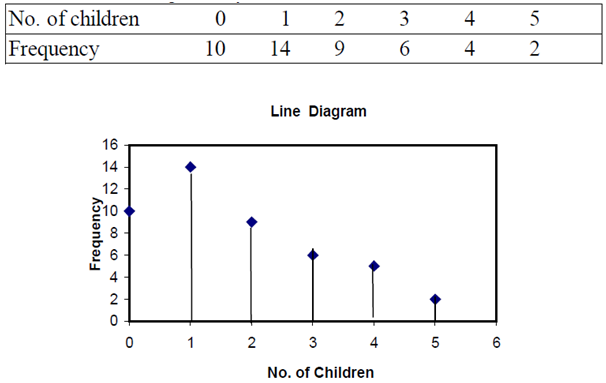### 2. Simple Bar Diagram:

Simple bar diagram can be drawn either on horizontal or vertical base, but bars on horizontal base more common. Bars must be uniform width and intervening space between bars must be equal. While constructing a simple bar diagram, the scale is determined on the basis of the highest value in the series.

To make the diagram attractive, the bars can be coloured.

Bar diagram are used in business and economics. However, an important limitation of such diagrams is that they can present only one classification or one category of data.

For example, while presenting the population for the last five decades, one can only depict the total population in the simple bar diagrams, and not its gender-wise distribution.

Example 2:

Represent the following data by a bar diagram.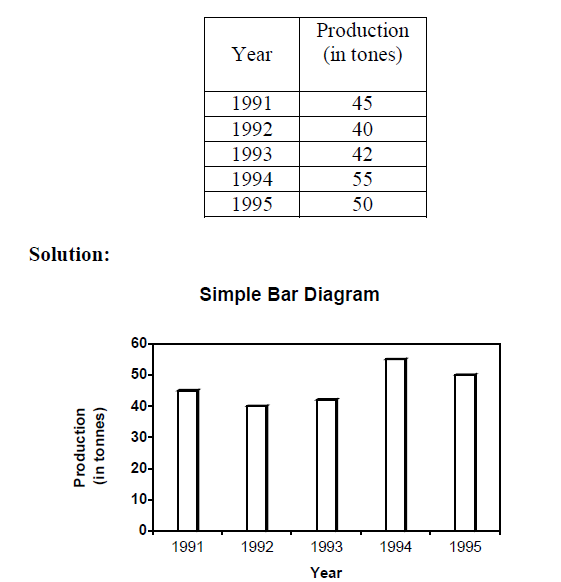### 3. Multiple Bar Diagram:

Multiple bar diagram is used for comparing two or more sets of statistical data. Bars are constructed side by side to represent the set of values for comparison. In order to distinguish bars, they may be either differently coloured or there should be different types of crossings or dotting, etc. An index is also prepared to identify the meaning of different colours or dotting.

Example 3:

Draw a multiple bar diagram for the following data.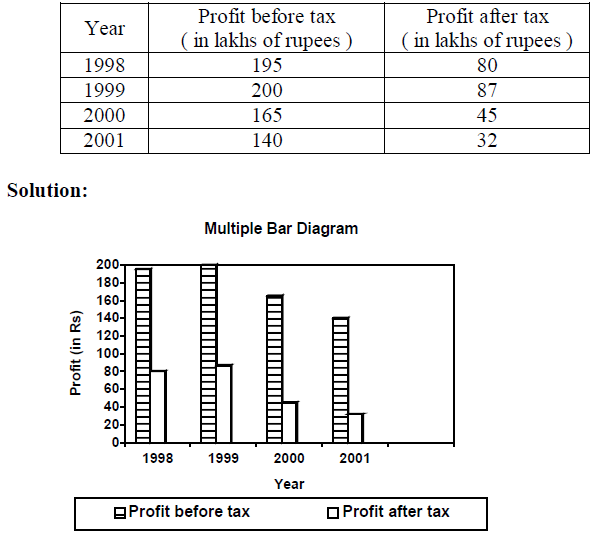### 4.Sub-divided Bar Diagram:

In a sub-divided bar diagram, the bar is sub-divided into various parts in proportion to the values given in the data and the whole bar represent the total. Such diagrams are also called Component Bar diagrams. The sub divisions are distinguished by different colours or crossings or dottings.

The main defect of such a diagram is that all the parts do not have a common base to enable one to compare accurately the various components of the data.

Example 4:

Represent the following data by a sub-divided bar diagram.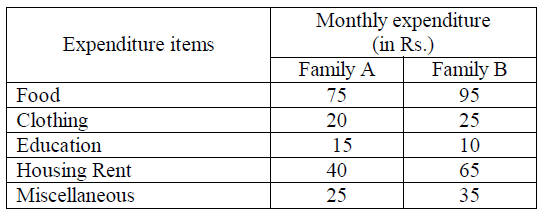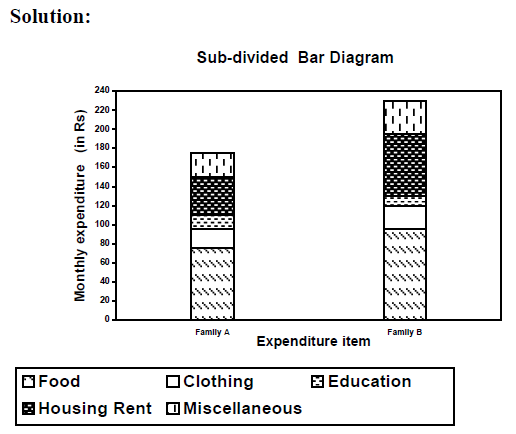### 5.Percentage bar diagram:

This is another form of component bar diagram. Here the components are not the actual values but percentages of the whole. The main difference between the sub-divided bar diagram and percentage bar diagram is that in the former the bars are of different heights since their totals may be different whereas in the latter the bars are of equal height since each bar represents 100 percent. In the case of data having sub-division, percentage bar diagram will be more appealing than sub-divided bar diagram.